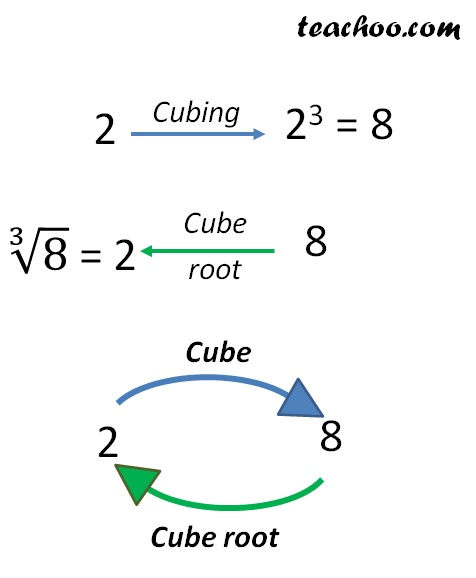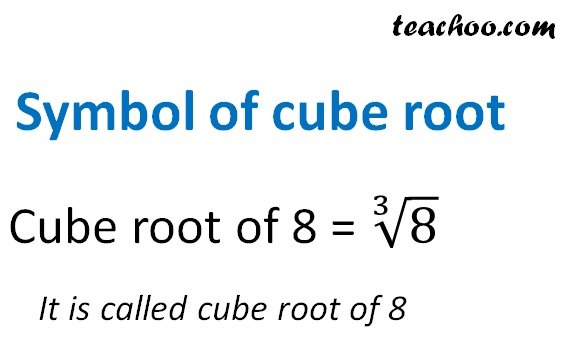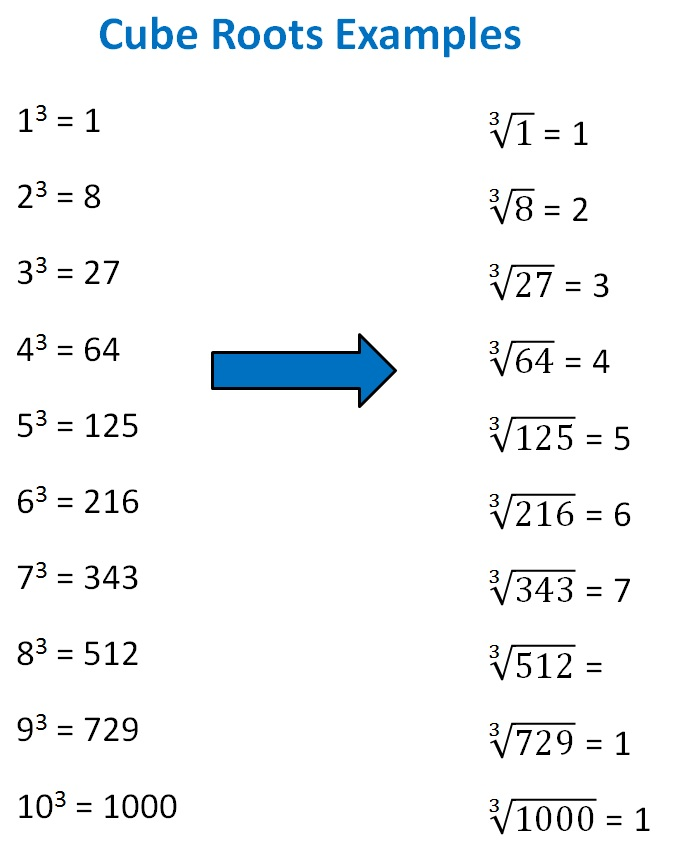Cube Root

Chapter 6 Class 8 Cubes and Cube Roots
Concept wise

Just like subtraction is the opposite of addition

Cube root is the opposite of cubing

Number = 2

Cube of number = 2 3

= 8

∴ Cube root of 8 = 2

We write ∛8 = 2Let’s look at cube roots of some numbersLearn in your speed, with individual attention - Teachoo Maths 1-on-1 Class